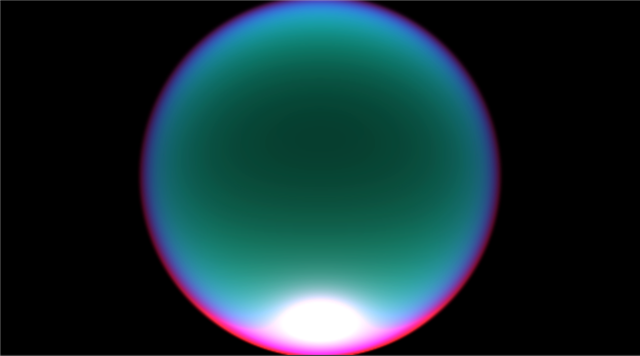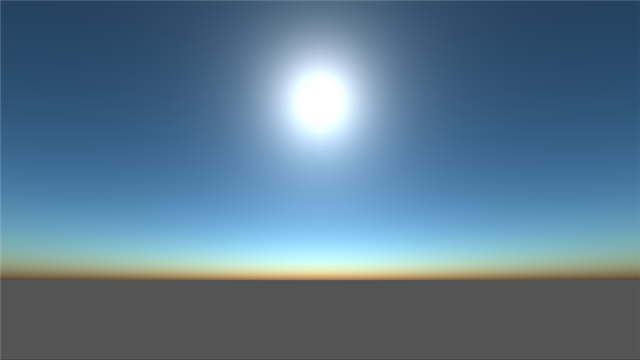# 大气渲染技术解析！如何在游戏中模拟出千变万化的天空？

1.1 介绍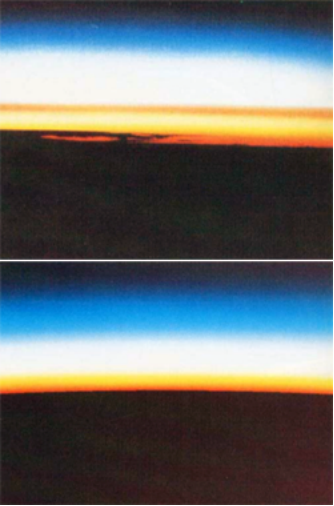Figure 1: Top, a real photograph of the Earth from outer space. Bottom, a simulation using Nishita's model. These images have been copied from the paper "Display of the Earth taking into account Atmospheric Scattering" written by Nishita in 1993 (c) Siggraph.

1.2 大气模型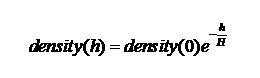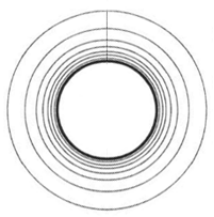Figure 2: aerosols present in the atmosphere are responsible for haze.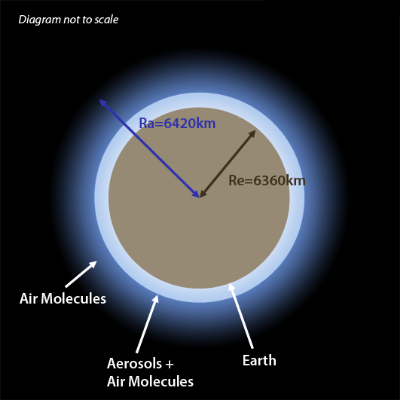Figure 3: a simple graphical representation of the atmospheric model we will be using in this lesson. It is defined by the radius of the planet (Re) and the radius of the atmosphere (Ra). The atmosphere is made of aerosols (mainly present at low altitude) and air molecules. Density of particles decreases exponentially with altitude. This diagram is not to scale.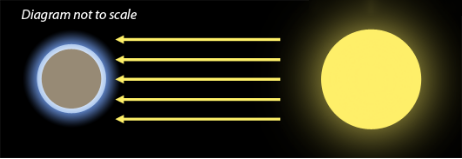Figure 4: the sun is so far away from the Earth, that each light ray reaching the Earth's atmosphere can be considered as being parallel to each other.

1.3 瑞利散射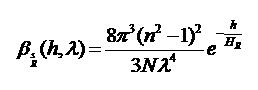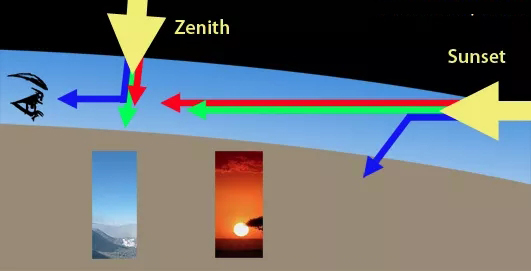Nishita的论文和我们在本篇中提到的其他论文的主要问题之一是他们要么没有给出N，n等的值来生成论文中显示的图像。在使用测量数据时，论文的作者通常不会引用它们的来源。在海平面上寻找分子密度的科学数据并不容易，如果你有任何关于此主题的有用链接或信息，你可以向我们提供，我们希望收到你的来信。我们在本篇中使用的海平面散射系数可以在两篇论文中找到："Efficient Rendering of Atmospheric Phenomena", by Riley et al. and "Precomputed Atmospheric Scattering" by Bruneton et al.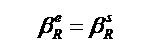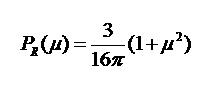1.4 米氏散射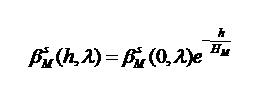1.5 光学深度的概念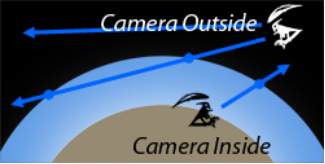Figure 6: the camera can either be inside the atmosphere or outside. When it is inside, we are only interested in the intersection point between the camera ray and the atmosphere. When the camera is outside it can intersect the atmosphere in two points.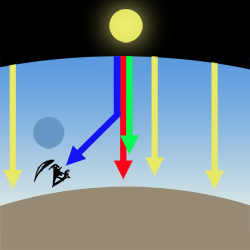Figure 7: single scattering is responsible for the sky color. A viewer is rarely directly looking at the sun (which is dangerous). However when we are looking away from the sun the atmosphere has a color which is the result of blue light from the sunlight being scattered in the direction of the observer's eyes.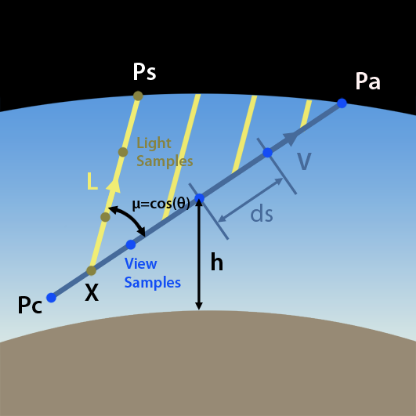Figure 8: to compute the sky color we first trace a ray from Pc to Pa and then integrate the amount of light coming from the sun (with direction L) that is reflected back along that ray (V).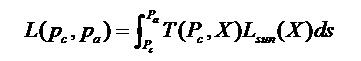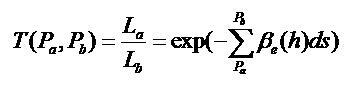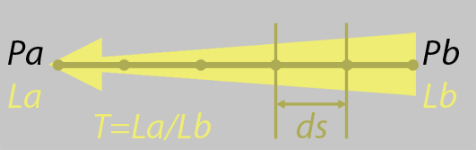Figure 9: as light (Lb) travels from Pb to Pa, it gets attenuated because of out-scattering and absorption. The result is La. Transmittance is the amount of light received at Pa from Pb, after it was attenuated while traveling through the atmosphere (that is T=La/Lb).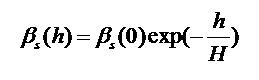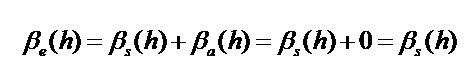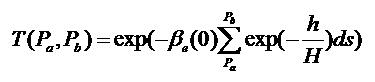1.6 将阳光加起来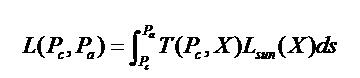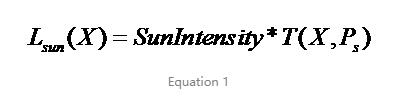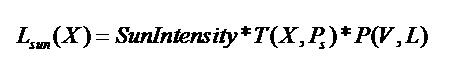1.7 计算天空颜色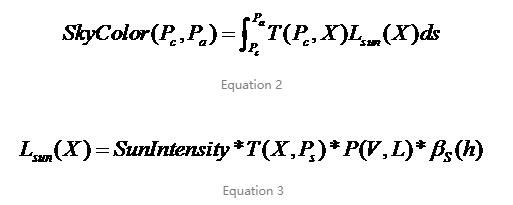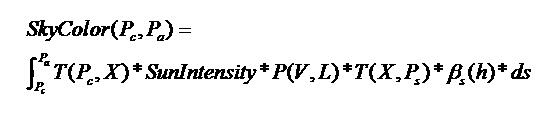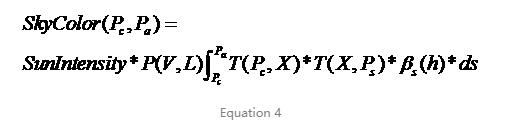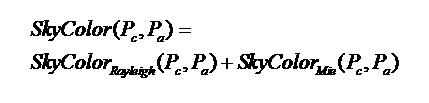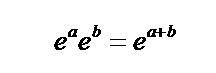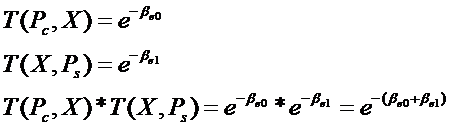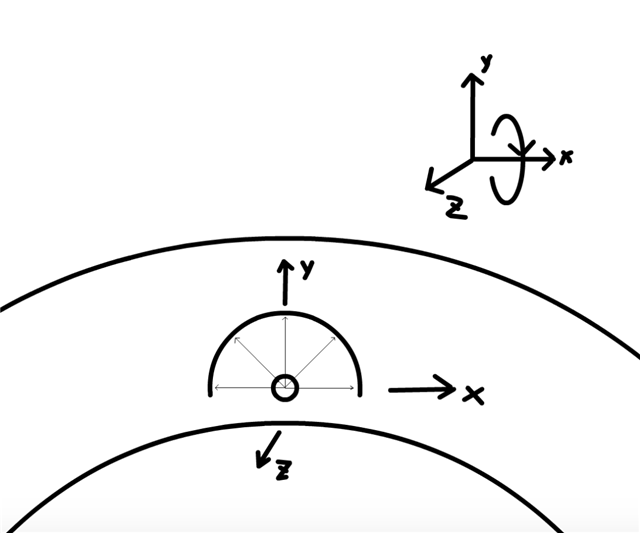vec2 uv =(2.*fragCoord/iResolution.xy1.)*vec2(iResolution.x/iResolution.y,1.);

float viewScale = 1.;

vec2 p = uv*viewScale;

//单位正方形内的点映射到半球面上

float z2 = p.x*p.x+p.y*p.y;

float phi = atan(p.y,p.x);

float theta = acos(1.-z2);

vec3 dir = vec3(sin(theta)*cos(phi),

cos(theta),

sin(theta)*sin(phi));

color = getIncidentLight(r);

fragColor = vec4(color,1.);

//sun position

float rotSpeedFactor = 3.;

mat3 sunRot = rotate_around_x(-abs(sin(u_time/rotSpeedFactor))*90.);

sunDir *= sunRot;

//绕x轴的旋转矩阵

mat3 rotate_around_x(const in float degrees)

{

float _sin = sin(angle);

float _cos = cos(angle);

return mat3(1.,0.,0.,

0.,_cos,-_sin,

0.,_sin,_cos);

}

#define PI 3.14159265359

#define USE_HENYEY 1

#define u_res iResolution

#define u_time iTime

vec3 sunDir = vec3(0.,1.,0.);

float sunPower = 20.;

const int numSamples = 16;

const int numSamplesLight = 8;//光线与大气层顶端交点到采样点的采样数量

const float hR = 7994.;//rayleigh

const float hM = 1200.;//mie

const vec3 betaR = vec3(5.5e-6,13.0e-6,22.4e-6);//rayleigh

const vec3 betaM = vec3(21e-6);//mie

struct ray

{

vec3 origin;

vec3 direction;

};

struct sphere

{

vec3 center;

};

//表示大气层的球

//计算射线与球是否相交以及交点

bool raySphereIntersect(const in ray r,const in sphere s,inout float t0,inout float t1)

{

vec3 oc = r.origin-s.center;

float a = dot(r.direction,r.direction);

float b = dot(oc,r.direction);

float discriminant = b*b-(a*c);

if(discriminant>0.0)

{

float tmp = sqrt(discriminant);

t0 = -b-tmp;

t1 = -b+tmp;

return true;

}

return false;

}

//----------------phase function----------------

float rayleighPhaseFunc(float mu)

{    return 3.*(1.+mu*mu)/(16.*PI);

}

const float g = 0.76;

float henyeyGreensteinPhaseFunc(float mu)

{

return (1.-g*g)/((4.*PI)*pow(1.+g*g-2.*g*mu,1.5));

}

const float k = 1.55*g-0.55*(g*g*g);

float schlickPhaseFunc(float mu)

{

return (1.-k*k)/(4.*PI*(1.+k*mu)*(1.+k*mu));

}

//----------------phase function----------------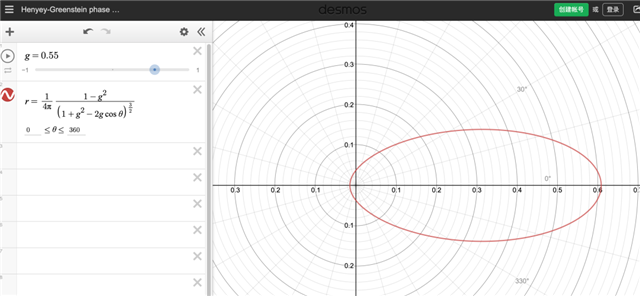//计算光学深度

bool getSunLight(const in ray r,inout float opticalDepthR,inout float opticalDepthM)

{

float t0,t1;

raySphereIntersect(r,atmosphere,t0,t1);

float marchPos = 0.;

float marchStep = t1/float(numSamplesLIght);

for(int i = 0;i

{

//相邻两个采样点的中点

vec3 s =r.origin+r.direction*(marchPos+0.5*marchStep);

if(height<0.)

return false;

opticalDepthR += exp(-height/hR)*marchStep;

opticalDepthM += exp(-height/hM)*marchStep;

marchPos += marchStep;

}

return true;

}

vec3 getIncidentLight(const in ray r)

{

float t0,t1;

if(!raySphereIntersect(r,atmosphere,t0,t1))

{

return vec3(0.);

}

float marchStep = t1/float(numSamples);

float mu = dot(r.direction,sunDir);

float phaseR = rayleighPhaseFunc(mu);

float phaseM =

#if USE_HENYEY

henyeyGreensteinPhaseFunc(mu);

#else

schlickPhaseFunc(mu);

#endif

float opticalDepthR = 0.;

float opticalDepthM = 0.;

vec3 sumR = vec3(0.);

vec3 sumM = vec3(0.);

float marchPos = 0.;

for(int i=0;i

{

vec3 s = r.origin+r.direction*(marchPos+0.5*marchStep);

//计算光学深度累加和

float hr = exp(-height/hR)*marchStep;

float hm = exp(-height/hM)*marchStep;

opticalDepthR += hr;

opticalDepthM += hm;

ray lightRay = ray(s,sunDir);

float opticalDepthLightR = 0.;

float opticalDepthLightM = 0.;

bool bOverGround = getSunLight(lightRay,opticalDepthLightR,opticalDepthLightM);

if(bOverGround)

{

vec3 t = betaR*(opticalDepthR+opticalDepthLightR)+

betaM*1.1*(opticalDepthM+opticalDepthLightM);

vec3 attenuation = exp(-t);

sumR += hr*attenuation;

sumM += hm*attenuation;

}

marchPos += marchStep;

}

return sunPower*(sumR*phaseR*betaR+sumM*phaseM*betaM);

}